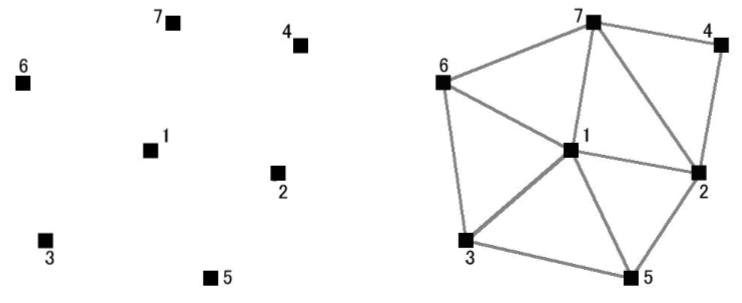603 - 5885 1250 (4 lines) info@mes100.com
Select Page

# Digital Terrain Method: Explanation and calculation

## DTM explanation#

1. Digital Terrain Model (DTM) is a digital representation of the continuous surface of ground by field survey points or data points with known X,Y,Z coordinates.
1. Main approach to terrain surface modelling is by Triangular Irregular Network (TIN) due to triangles is the most basic unit in all geometrical patterns and have great flexibility in terms of shape & size.1. In TIN, ‘best fit’ triangles are formed between data points. ‘Best fit’ triangle is formed with nearest neighbor points, that is the sum of three edges of the triangle is the minimum (Delaunay Triangulation ).
2. And then, the meshed triangle will be overlayed on the platform, in order to form individual Polyhedron bounded by the platform level and the terrain level. Each of the volume of Polyhedron will be calculated. If the terrain level is higher than platform level, then it is a cut. If the terrain level is lower than platform level, then it is a fill.

## DTM Examples#

#### Surveyor points#

Assuming that we have a group of surveyor points:
n0 (0,0,0)
n1(10,0,0)
n2(10,10,0)
n3(0,10,0)
n4(5,5,10)
Then 4 element mesh will be formed,

1. Ea Consist of n0, n1, n4
2. Eb Consist of n1, n2, n4
3. Ec Consist of n2, n3, n4
4. Ed Consist of n0, n3, n4

#### Platform#

Assuming that we now have a platform of the following points:
n5 (0,0,-5)
n6(10,0,-5)
n7(10,10,-5)
n8(0,10,-5)
We add a new point n9(5,5,-5)for later purpose

Then in DTM, the surveyor point mesh will be superimposed on the platforms, resulting in polyhedrons that can be used to calculate cut/fill individually.

1. PaConsist of n0,n1,n4, n5,n6,n9
2. PbConsist of n1,n2,n4, n6,n7,n9
3. PcConsist of n2,n3,n4, n7,n8,n9
4. PdConsist of n3,n4,n0, n8,n5,n9

An illustration is shown below

## Volume Calculation#

To calculate volume the Pa can be further broken into two parts:
Part 1, with flat on both top and bottom side
Part 2, with flat on bottom side, and a slanted triangle on the top side
The volume for
Part 1 is equal to V=area*height=1/2*(10*5)*5=125
Part 2 is equal to V=1/3 * area*height=1/3*((1/2*10*5)*10)=83.33
Thus the volume for Pa is 125+83.33=208.33

Sum up all the volumes for Pa, Pb, Pc, Pd and you will get the total volume. In this case, since the ground level is higher than platform level, then it is a cut volume.

The 3D drawing is as below:

The polyhedron is divided into 4

Part a is divided into two; Part 1 and Part 2

We also carry out an example to benchmark the calculation with our software. The discrepancy of the calculation is also calculated in the example, which is less than 1%. The slight discrepancy is due to the finite precision approximation algorithm of snap rounding.

We also provide you the example of the project file for you to refer (The example is using MiTS 2.1.50.0, if users are not able to open the project, kindly contact MES support team).

The result obtained in the software is more less the same, as stated in the benchmark, which is 830.82 m3 in MiTS and 833.33 m3 in the benchmark, with discrepancy of 0.3%.